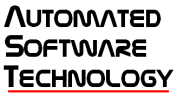# LabVIEW

cancel
Showing results for
Did you mean:

## Calculate sinusoidal Position from acceleration data

Hello,

Hopefully this is an easy answer.  I have a sinusoidal waveform and I want to take the double integral of it.  i.e. I have acceleration and I want position.  When I do it in LabVIEW I get weird results.  Why isn't my position sinusoidal?  What am I doing wrong?

ThanksDan Shangraw, P.E.Message 1 of 18
(279 Views)

## Re: Calculate sinusoidal Position from acceleration data

"C"

There is a "C" in both of those integrals.

Ben

Message 2 of 18
(271 Views)

## Re: Calculate sinusoidal Position from acceleration data

Another way to look at it, is that the velocity is always positive, so the distance / position is always increasing.

Message 3 of 18
(264 Views)
Highlighted

## Re: Calculate sinusoidal Position from acceleration data

As Sir Ben said, small offsets in data will lead to wild swings when you do the integral. A few solutions:

1. Remove mean from data, then high pass filter with a cutoff of 1 Hz, then integrate.
2. Take the frequency spectrum, excluding 0 frequency divide each frequency by (2 pi f) for v or (2 pi f) for d. Measure displacements in frequency space.

mcduff

EDIT: (2 pi f)^2 for d

Message 4 of 18
(252 Views)

## Re: Calculate sinusoidal Position from acceleration data

Hello,

Yes I have looked up the calculus and saw the C.  Its been a while...

A little more background my customer has an accelerometer that we are acquiring data from.  They want to know the displacement.  Performing a double integral on the accelerometer data should give me the displacement.  I am expecting a sinusoidal displacement signal.  That is how the UUT is actually moving.  The accelerometer data is sinusoidal.  I made this quick VI to test my math.  I am confused at the results I am getting and what math I need to calculate displacement.

Thanks

Dan Shangraw, P.E.Message 5 of 18
(247 Views)

## Re: Calculate sinusoidal Position from acceleration data

Hello mcduff,

What does remove mean refer to?  DC?  Shouldn't the High Pass filter get that too?

Dan Shangraw, P.E.Message 6 of 18
(246 Views)

## Re: Calculate sinusoidal Position from acceleration data

@ASTDan wrote:

Hello mcduff,

What does remove mean refer to?  DC?  Shouldn't the High Pass filter get that too?

Yes and Yes, I like to do both even though it may not be necessary. Doing the calculation in frequency space is much easier, no filters or DC removal.

mcduff

Message 7 of 18
(243 Views)

## Re: Calculate sinusoidal Position from acceleration data

It sounds like you are expecting a harmonic solution, but you are not providing the proper boundary condition.  Using the default initial condition for the velocity (1st integral), implies v(0) = 0.  For the harmonic solution, v = 0 at the extrema of the acceleration, so if you set the phase of your acceleration waveform to 90 (or 270) degrees you should see what you are expecting.

Message 8 of 18
(241 Views)

## Re: Calculate sinusoidal Position from acceleration data

Also keep in mind there is no such thing as a "zero" in real world measurements.

And even if you found the offset, real-word values drift ever so slightly and they will pile up and you are right back where you started, trying to figure what the correct "C" should be.

Ben

Message 9 of 18
(233 Views)

## Re: Calculate sinusoidal Position from acceleration data

Changing that phase is better.  However how do I set the initial conditions?  I don't have the luxury of manipulating phase to get the desired results.  I set the initial conditions in the integration VIs but still don't get expected results when using 0 phaseDan Shangraw, P.E.